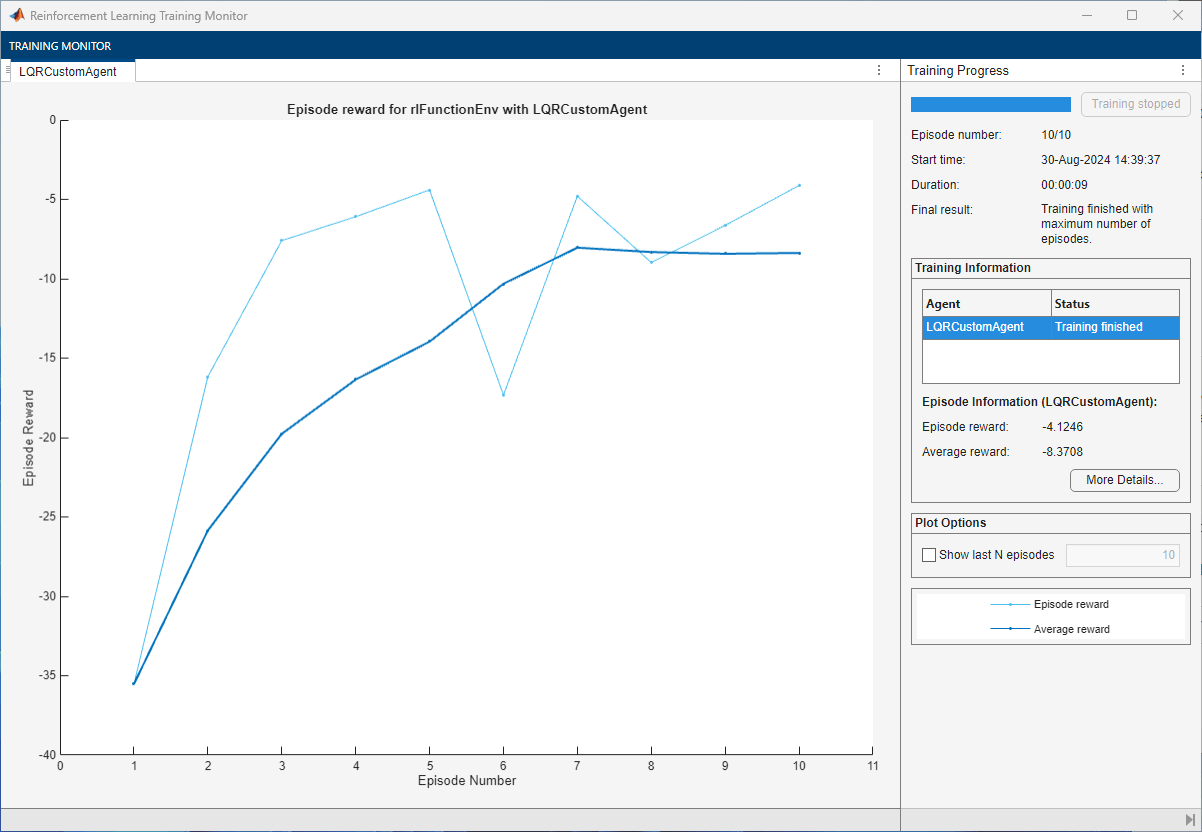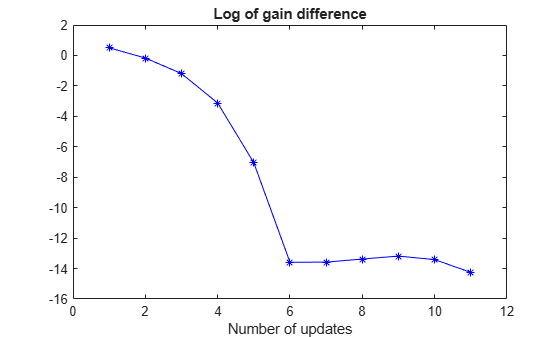# Create and Train Custom LQR Agent

This example shows how to create and train a custom linear quadratic regulation (LQR) agent to control a discrete-time linear system modeled in MATLAB®. For a introduction to custom agents, see Create Custom Reinforcement Learning Agents. For a step by step example on how to create a custom PG agent (using the REINFORCE algorithm) see Create and Train Custom PG Agent. For an example of how a DDPG agent can be used as an optimal controller for a discrete-time system, see Compare DDPG Agent to LQR Controller.

### Create Linear System Environment

The reinforcement learning environment for this example is a discrete-time linear system. The dynamics for the system are given by

`${x}_{t+1}=A{x}_{t}+B{u}_{t}$`

The feedback control law is

`${u}_{t}=-K{x}_{t}$`

The control objective is to minimize the quadratic cost: $\mathit{J}={\sum }_{\mathit{t}=0}^{\infty }\left({{\mathit{x}}_{\mathit{t}}}^{\prime }{\mathit{Qx}}_{\mathit{t}}+{{\mathit{u}}_{\mathit{t}}}^{\prime }{\mathit{Ru}}_{\mathit{t}}\right)$.

In this example, the system matrices are

`$\begin{array}{l}\mathit{A}=\left[\begin{array}{ccc}1.05& 0.05& 0.05\\ 0.05& 1.05& 0.05\\ 0& 0.05& 1.05\end{array}\right]\\ \mathit{B}=\left[\begin{array}{ccc}0.1& 0& 0.2\\ 0.1& 0.5& 0\\ 0& 0& 0.5\end{array}\right]\end{array}$`

```A = [1.05,0.05,0.05;0.05,1.05,0.05;0,0.05,1.05]; B = [0.1,0,0.2;0.1,0.5,0;0,0,0.5]; ```

`$\begin{array}{l}\mathit{Q}=\left[\begin{array}{ccc}10& 3& 1\\ 3& 5& 4\\ 1& 4& 9\end{array}\right]\\ \mathit{R}=\left[\begin{array}{ccc}0.5& 0& 0\\ 0& 0.5& 0\\ 0& 0& 0.5\end{array}\right]\end{array}$`

```Q = [10,3,1;3,5,4;1,4,9]; R = 0.5*eye(3);```

For this environment, the reward at time $\mathit{t}$ is given by ${r}_{t}=-{x}_{t}"Q{x}_{t}-{u}_{t}"R{u}_{t}$, which is the negative of the quadratic cost. Therefore, maximizing the reward minimizes the cost. The initial conditions are set randomly by the reset function.

Create the MATLAB environment interface for this linear system and reward. The `myDiscreteEnv` function creates an environment by defining custom `step` and `reset` functions. For more information on creating such a custom environment, see Create Custom Environment Using Step and Reset Functions.

`env = myDiscreteEnv(A,B,Q,R);`

Fix the random generator seed for reproducibility.

`rng(0)`

### Create Custom LQR Agent

For the LQR problem, the Q-value function for a given control gain $\mathit{K}$ is quadratic and can be defined as ${\mathit{Q}}_{\mathit{K}}\left(\mathit{x},\mathit{u}\right)={\left[\begin{array}{c}\mathit{x}\\ \mathit{u}\end{array}\right]}^{\prime }{\mathit{H}}_{\mathit{K}}\left[\begin{array}{c}\mathit{x}\\ \mathit{u}\end{array}\right]$, where ${\mathit{H}}_{\mathit{K}}=\text{\hspace{0.17em}}\left[\begin{array}{cc}{\mathit{H}}_{\mathrm{xx}}& {\mathit{H}}_{\mathrm{xu}}\\ {\mathit{H}}_{\mathrm{ux}}& {\mathit{H}}_{\mathrm{uu}}\end{array}\right]$ is a symmetric, positive definite matrix.

The control law that maximizes ${\mathit{Q}}_{\mathit{K}}$ is $\mathit{u}=-{\left({\mathit{H}}_{\mathrm{uu}}\right)}^{-1}{\mathit{H}}_{\mathrm{ux}}\text{\hspace{0.17em}}\mathit{x}$, so the feedback gain is ${\mathit{K}=-\left({\mathit{H}}_{\mathrm{uu}}\right)}^{-1}{\mathit{H}}_{\mathrm{ux}}\text{\hspace{0.17em}}$.

The matrix ${\mathit{H}}_{\mathit{K}}$ contains $\mathit{m}=\frac{1}{2}\mathit{n}\left(\mathit{n}+1\right)$ distinct element values, where $\mathit{n}$ is the sum of the number of states and number of inputs. Denote $\theta$ as the vector containing these $\mathit{m}$ elements, in which the off-diagonal elements in ${\mathit{H}}_{\mathit{K}}$ are multiplied by two. The elements of $\theta$ are the parameters that the custom agent needs to learn.

You can express the Q-value function as the inner product of the vectors $\theta$ and $\varphi \left(\mathit{x},\mathit{u}\right)$: ${\mathit{Q}}_{\mathit{K}}\left(\mathit{x},\mathit{u}\right)={\theta }^{\prime }\varphi \left(\mathit{x},\mathit{u}\right)$, where $\varphi \left(\mathit{x},\mathit{u}\right)$ is a vector of quadratic monomials built from the combination of all the elements in $\mathit{x}$ and $\mathit{u}$. For an example, see the $\mathit{Q}\left(\mathit{x},\mathit{u}\right)$ matrix in Compare DDPG Agent to LQR Controller.

The LQR agent starts with a stabilizing controller ${\mathit{K}}_{0}$. To get an initial stabilizing controller, place the poles of the closed-loop system $\mathit{A}-{\mathit{BK}}_{0}$ inside the unit circle.

`K0 = place(A,B,[0.4,0.8,0.5]);`

To create a custom agent, you must create a subclass of the `rl.agent.CustomAgent` abstract class. For the custom LQR agent, the defined custom subclass is `LQRCustomAgent`. For more information, see Create Custom Reinforcement Learning Agents. Create the custom LQR agent using $\mathit{Q}$, $\mathit{R}$, and ${\mathit{K}}_{0}$. The agent does not require information on the system matrices $\mathit{A}$ and $\mathit{B}$.

`agent = LQRCustomAgent(Q,R,K0);`

For this example, set the agent discount factor to one. To use a discounted future reward, set the discount factor to a value less than one.

`agent.Gamma = 1;`

Because the linear system has three states and three inputs, the total number of learnable parameters is $\mathit{m}=21$. To ensure satisfactory performance of the agent, set the number of parameter estimates ${\mathit{N}}_{\mathit{p}}$ (the number of data point to be collected before updating the critic) to be greater than twice the number of learnable parameters. In this example, the value is ${\mathit{N}}_{\mathit{p}}=45$.

`agent.EstimateNum = 45;`

To get good estimation results for $\theta$, you must apply a persistently excited exploration model to the system. In this example, encourage model exploration by adding white noise to the controller output: ${\mathit{u}}_{\mathit{t}}=-{\mathit{Kx}}_{\mathit{t}}+{\mathit{e}}_{\mathit{t}}$. In general, the exploration model depends on the system models.

### Train Agent

To train the agent, first specify the training options. For this example, use the following options.

• Run each training episode for at most 10 episodes, with each episode lasting at most 50 time steps.

• Display the training progress in the Episode Manager dialog box (set the `Plots` option) and disable command line display (set the `Verbose` option).

For more information, see `rlTrainingOptions`.

```trainingOpts = rlTrainingOptions(... MaxEpisodes=10, ... MaxStepsPerEpisode=50, ... Verbose=false, ... Plots="training-progress");```

Train the agent using the `train` function.

`trainingStats = train(agent,env,trainingOpts);`### Simulate Agent and Compare with Optimal Solution

To validate the performance of the trained agent, simulate it within the MATLAB environment. For more information on agent simulation, see `rlSimulationOptions` and `sim`.

```simOptions = rlSimulationOptions(MaxSteps=20); experience = sim(env,agent,simOptions); totalReward = sum(experience.Reward)```
```totalReward = -30.6482 ```

You can compute the optimal solution for the LQR problem using the `dlqr` function.

`[Koptimal,P] = dlqr(A,B,Q,R); `

The optimal reward is given by ${\mathit{J}}_{\mathrm{optimal}}={{-\mathit{x}}_{0}}^{\prime }{\mathit{Px}}_{0}$.

```x0 = experience.Observation.obs1.getdatasamples(1); Joptimal = -x0'*P*x0;```

Compute the error in the reward between the trained LQR agent and the optimal LQR solution.

`rewardError = totalReward - Joptimal`
```rewardError = 5.0439e-07 ```

View the history of the norm of the difference between the gains between the trained LQR agent and the optimal LQR solution.

```% Number of gain updates len = agent.KUpdate; % Initialize error vector err = zeros(len,1); % Fill elements for i = 1:len err(i) = norm(agent.KBuffer{i}-Koptimal); end % Plot logarithm of the error vector plot(log10(err),'b*-') title("Log of gain difference") xlabel("Number of updates")```Compute the norm of final error for the feedback gain.

`gainError = norm(agent.K - Koptimal)`
```gainError = 1.6756e-11 ```

Overall, the trained agent finds a solution that is very close to the true optimal LQR solution.